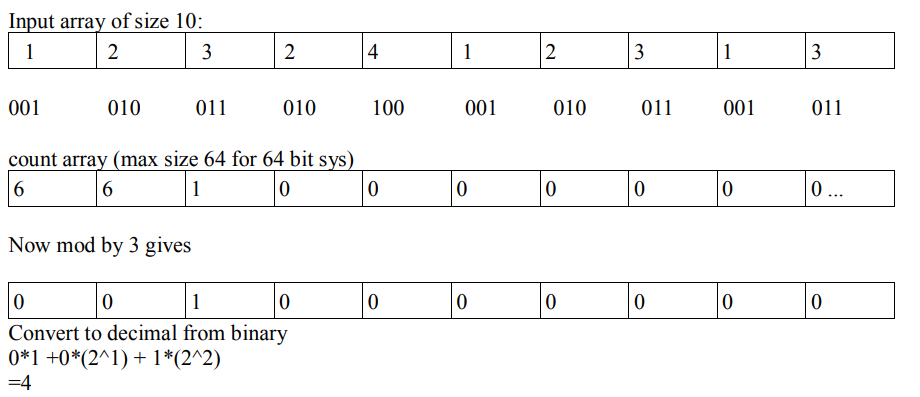Q:

# C++ program to find Unique Number using Bit Masking

C++ Program to find unique number in an array of n numbers in which except one (unique number) rest all are present thrice.

Constraints: n<10^5

Example:

```    Input:
10
1  2  3  2  4  1  2  3  1  3

Output:
4
```

Solution: Except 4 rest all have multiplicity of 3 in array. For instance 4 are represented as 100 in binary .Starting from left most bit is the first index of count array.Program:

``````#include <iostream>
using namespace std;

int unique(int *arr,int n)
{
//array of size 64 for max 64 bit size
int count={0};

//count array stores bit of every number
for(int k=0;k<n;k++)
{
int i=0;
int num=arr[k];
while(num>0)
{
// extract bit
count[i]+=(num&1);
i++;
// right shift to get next leftmost bit
num=num>>1;
}
}

// starting from first index 2^0=1
int power=1;
int result=0;
for(int j=0;j<64;j++)
{
// take modulus of count array by 3
count[j] %=3;
result+=count[j]*power;
// binary to decimal operation
power=power<<1;
}
// if there is no unique number 0 is returned
return result;
}

int main()
{
int arr;
int n;
cout<<"Enter lenght of the array: ";
cin>>n;

cout<<"Enter array elements..."<<endl;
for(int c=0;c<n;c++)
{
cin>>arr[c];
}
cout<<unique(arr,n)<<" is the unique number in array.";

return 0;
}
``````

Output

```Enter lenght of the array: 4
Enter array elements...
10 10 10 40
40 is the unique number in array.```